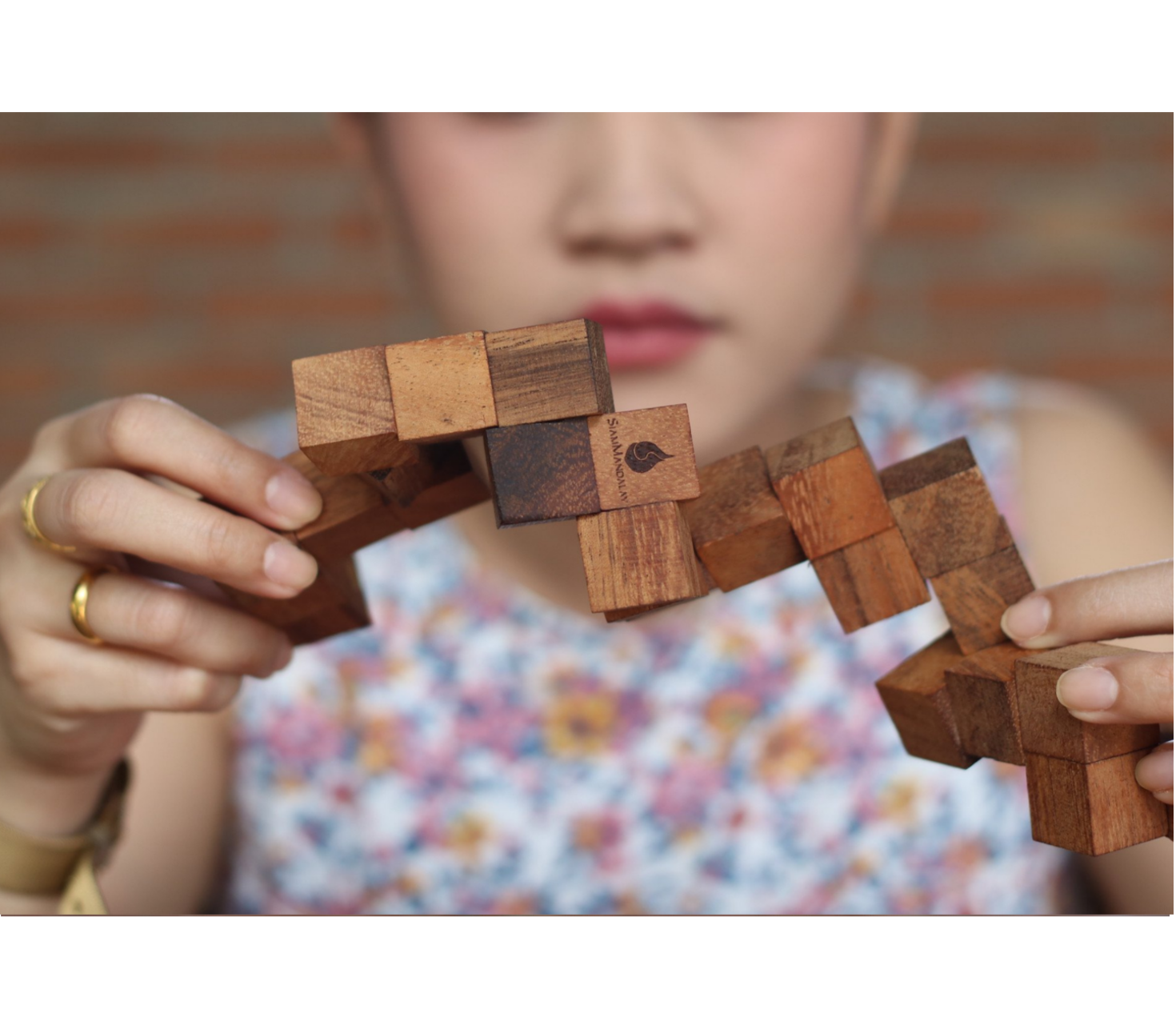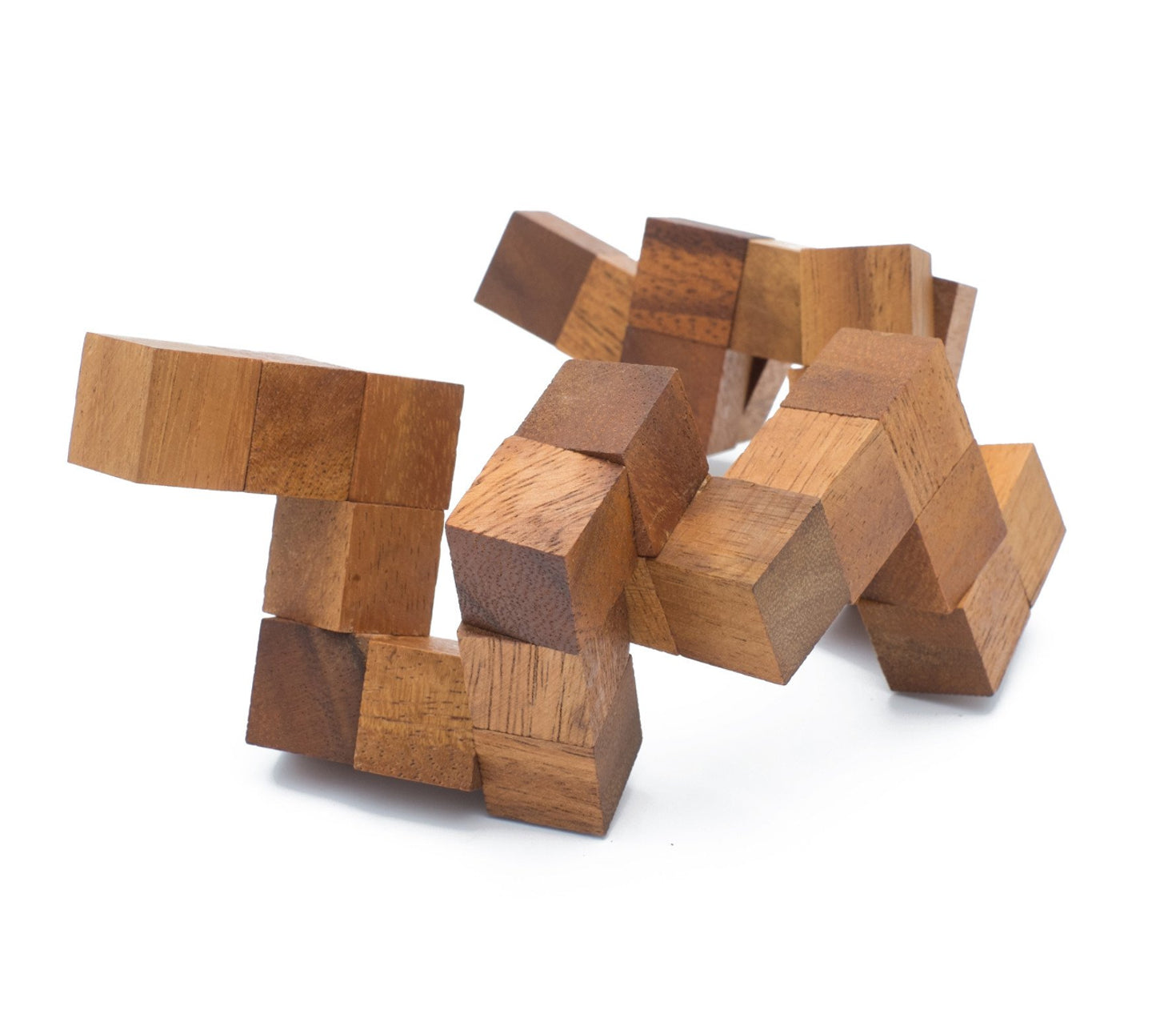HOW TO SOLVE A SNAKE CUBE PUZZLE | STEP BY STEP SOLUTIONS IN 3D

The snake cube puzzle is a puzzle of cubes that are connected with an elastic band that passes through their middle. Ideally, a snake cube puzzle should have 27 or 64 cubes. In recent times there has been an emergence of similar puzzles with a higher number of cubes.Each cube, apart from those on either end, has two faces that have holes through their centre. While some cubes have holes in opposite faces thus making the elastic band run straight through them, others have them on adjacent faces, thus making the elastic band make a 90º turn. This allows for easy rotation and twisting as you seek a serpent cube solution.There are quite some versions with the most popular being the wooden snake cube puzzle solution. Some of these puzzles are easier than others with the easiest ones being the straight ones being the easiest. Other versions have multiple solutions. Still, some versions have only a single solution.

In this case, we will be discussing how to solve the 27-cubed puzzle. When unsolved, the puzzle is a strip of 17 groups with two or three cubes each. The aim is to arrange the strip in such a way that in the end, there is a 3x3x3 cube.

## How to solve the puzzle – step by step snake cube puzzle instructions

1. Look for cube sections that have the number ‘3’ as they form the starting point for you to solve the puzzle successfully.

2. The first move is to align Two ‘3’ cube sections so that they are alongside one another.

3. Stand up the two ‘3’ cube sections so that they are now upright. Flip it so that cubes adjacent to the already upright portion are on the table’s side rather than dangling like the rest of the unsolved strip.

4. Rotate the cubes in the ‘L’ shape for three times to complete the base of the cube.

5. From here on, it becomes pretty easy as it’s just a matter of wrapping the remaining strand around the core and filling it as you continue. This is accomplished by rotating, twisting, and filling.

6. The final move for the cube’s top is in the ‘U’ shape, after which you just rotate and snap the remaining strip in place.

## General hints on how to solve a snake cube

If the snake cube puzzle alternate in colour, it is clear that one colour has one extra cube. One colour will form the face centres and the corners. Either end of the cube must, therefore, be at the centre or the corners.
If the snake puzzle has several straight strips close to each other, try starting with these sections first.

If the puzzle has a straight part that is of the same colour, it follows that this part should lie at the centre. It would be ideal to start solving it from there.

The snake cube puzzle should not be confused with the Kibble cube. Though they almost resemble one another, in the latter, the elastic band can enter or exit different faces of the cubes. When making the large cube, the colours on its sides have to match as well, which is not the case when solving a snake cube puzzle.

Dezember 23, 2014 —